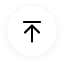# Free Editable Problem and Solution Graphic Organizer Examples

Problem and solution diagrams are useful tools that can be used in order to describe an issue or problem along with proposed steps for its elimination as well as a final solution at the end. If you are looking to understand more about problem and solution graphic organizers, keep on reading till the end. In this post, we are going to touch up some base concepts about these organizers while explaining some examples along the way.

## 1. What is a Problem and Solution Graphic Organizer

In summation, a problem and solution graphic organizer is defined as a graphical representation of a problem-solving process. The diagram involves writing the essence of the problem in the beginning, then suggesting steps for resolving it, and finally coming up with a solution. By using these diagrams, it becomes easy for a person or team to organize the relevant information into an easily perusable and understandable form.

The reason why problem and solution diagrams are so important is that they are easy to read and present. Should an organization face a problem or issue, the relevant team or department can come up with a simple and straightforward graphic organizer that depicts the best route forward while enumerating the steps necessary for taking that certain route.

If there is any modification that needs to be made with a certain aspect of the solution, it can be easily done so since every step will be separately and clearly mentioned.

## 2. The Problem and Solution Graphic Organizer Examples

Problem and solutions graphic organizers are used for depicting the steps needed for reconciliation when a certain problem arises. If two or more parties are involved in the resolution of any issue, it is important for them to be on the same level of understanding. Similarly, if there is a large organization and there are a lot of people who need to take part in solving the problem, it is essential that each one of them knows and understands what the issue is, what the steps are that are needed for the resolution and what the final solution will be. By using a problem and solution graphic organizer, you can delegate that knowledge and teach a large number of people the protocol that needs to be followed when solving a problem. The graphic organizer can be presented at a meeting or it can be individually given to each of the involved personnel.

Here are some examples of problem and solution diagrams:

##### Example 1: Problem and Solution Graphic Organizer

This graphic organizer depicts a simple problem and solution layout. In this example, the problem is shown at the top of the page and it is divided into three sections viz. ‘Who?’, ‘What?’ and ‘Why?’

The benefit of this segregation is that it not only helps the user to define the problem, but it also enables them to describe who the problem pertains to, what the problem actually is and why the problem surfaced. The simple method would be to mention the problem all in one go but dividing them like this gives greater clarity into the matter.

Then comes the ‘Possible Solutions’ section. This is where all possible solutions to the problem are discussed and brainstorming is done. After that, the selected/most suitable solution is delineated in the last section.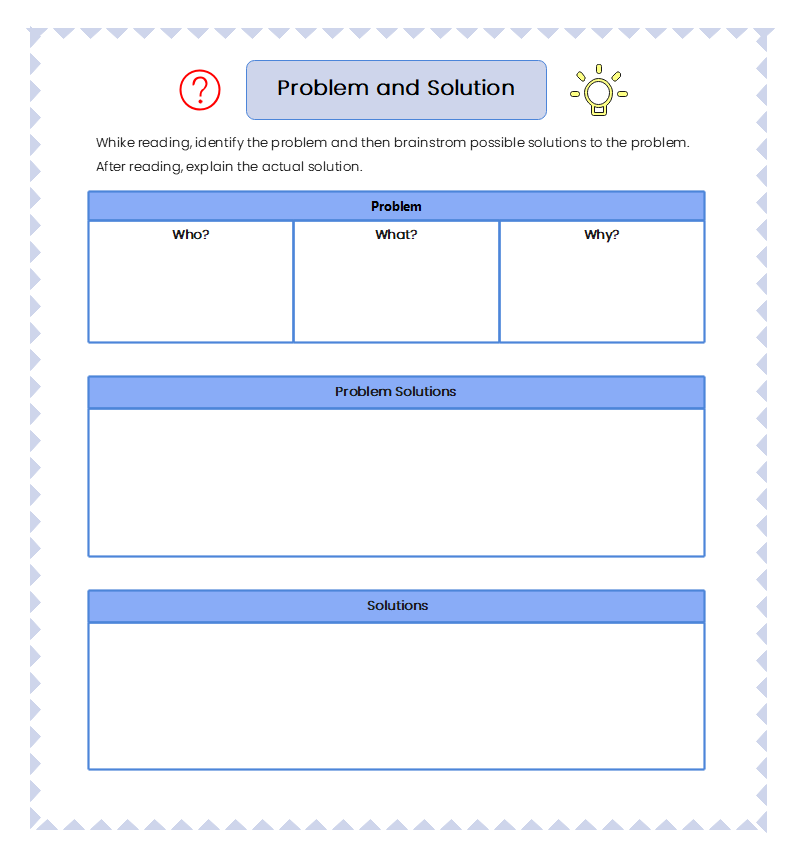Source: EdrawMax Online Edit Now

##### Example 2: Weekly Problem and Solution Graphic Organizer

In this example, the layout is a little different. This organizer depicts a problem-solving sheet for five days of a week viz. from Monday to Friday. Each day has three columns in front of it which are for "Rough Work/Drawing", "Sum/Number Sentence" and "Answer" respectively. Instead of focusing on a problem and giving a solution to it (as was the case in the previous example), this organizer features a set of columns and rows designed for students to help with their weekly problem-solving. This is namely an activity sheet for kids rather than an actual problem-solving diagram that can be officially used etc. This template can be useful to accomplish something similar to what it is made for i.e. learning and teaching, but it is not suitable for using to depict a certain problem and its solution.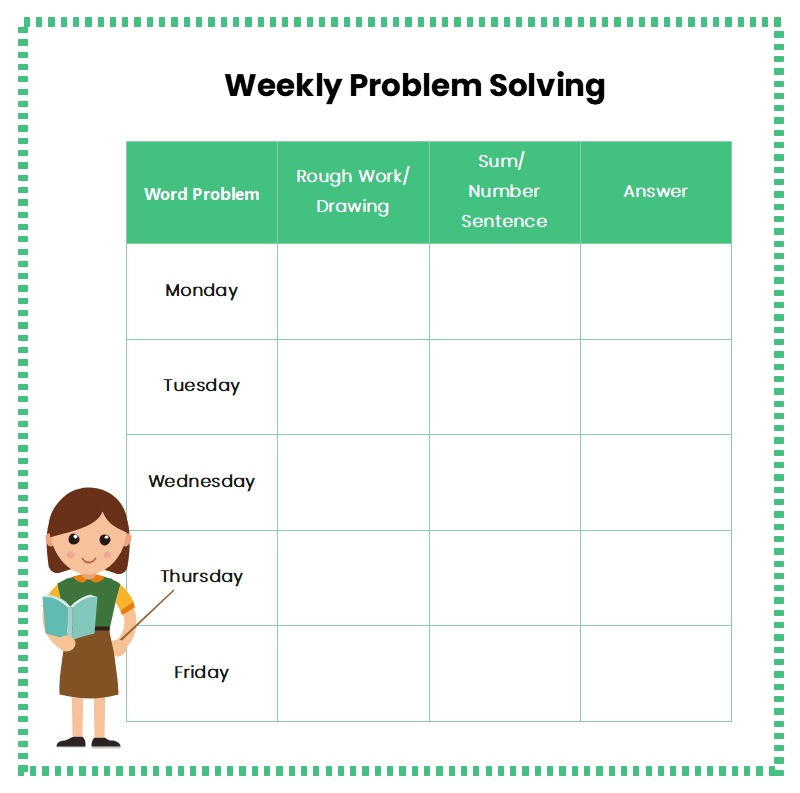Source: EdrawMax Online Edit Now

##### Example 3: Problem & Solution Graphic Organizer

The Problem & Solution Graphic Organizer features a simple problem-solving structure. In this diagram, three boxes on the left are made for the problems while three boxes adjacent to them are for their respective solutions. This design does not have the sequence where the problem first appears followed by the steps of resolution and then the solution. Here, three problems are simultaneously listed with their solutions written directly beside them without any ‘Possible Solutions’ section or steps for resolution.

This sort of graphic organizer can be useful in circumstances where a multitude of problems have arisen at the same time and need to be dealt with swiftly. In such an instance, listing possible alternatives or steps can be arduous, and simply writing the solutions can be much easier.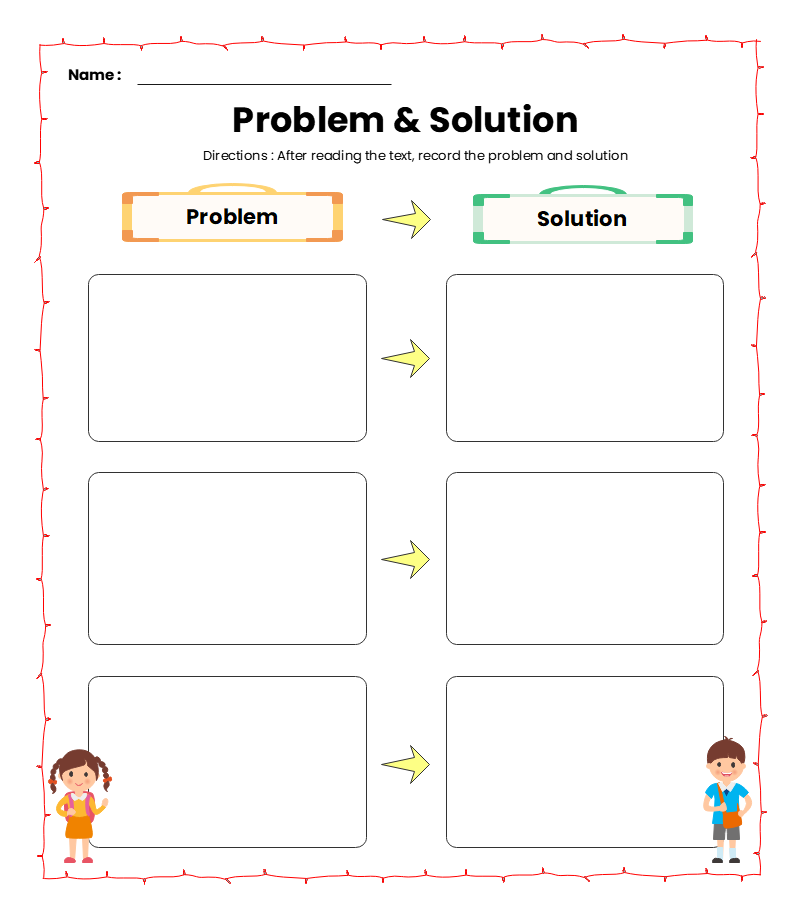Source: EdrawMax Online Edit Now

##### Example 4: Problem Solution Graphic Organizer

This example features an unusual layout that can be used for problem-solving. Instead of listing the problem, the steps/possible solutions, and then lastly the solution, this example first mentions the problem and then three 'Goals' in the form of 'Event # 1, 2, and 3'. In the 'Event' spaces, you can put in the outcomes you require or the situations you need to find yourself in after resolving the problem. Hence, you would be enumerating the goals you need to achieve by solving the problem.

After the ‘Goals’, the ‘Resolution’ section is given. In this section, one could write the actual steps or procedure that needs to be followed to meet the above ‘Goals’. This template can be more suitable for intricate issues rather than simple problems that require a one-line solution.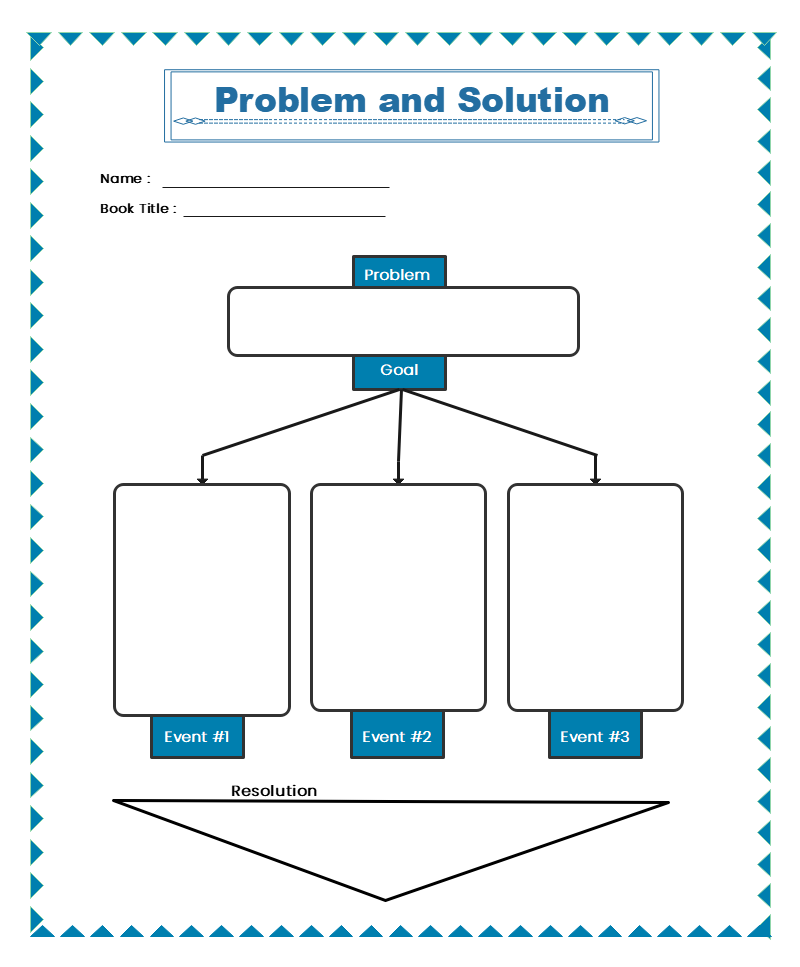Source: EdrawMax Online Edit Now

##### Example 5: Problem and Solution Graphic Organizer Example

In this example, a very practical and useful approach is used. As we saw before, enumeration of possible solutions is useful for brainstorming and selecting the best one of the lot. This example makes it really easy and simple to compare alternative solutions. It first depicts the problem at the start of the page. Then, three choices are listed underneath the problem. For each choice, a ‘Pros/Cons’ list is given. Finally, in the end, there is a 'Solution' section where you can write the choice you selected and why it is the best one.

By listing the pros and cons of each choice, it can be established which route or plan of action is the most suited and beneficial. The choice which has the most pros and least number of cons can be easily selected.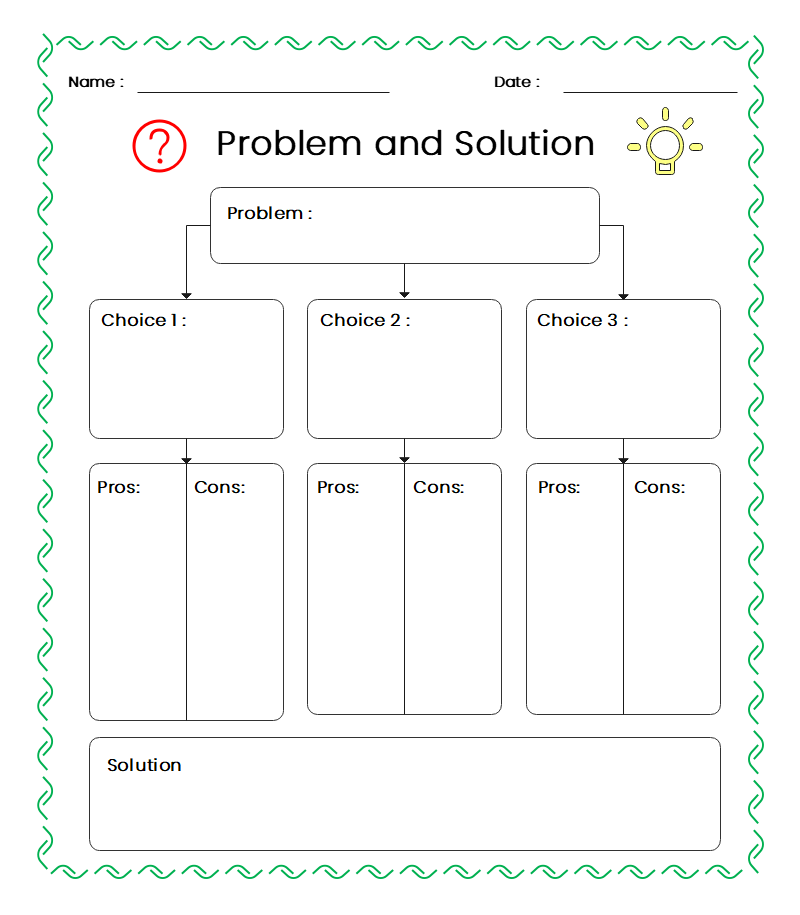Source: EdrawMax Online Edit Now

##### Example 6: Problem and Solution Graphic Organizer PDF

This graphic organizer is labeled 'Compare and Contrast'. In this example, there are two different sets of problems and solutions. Each set starts off with the 'Text', succeeded by the 'Problem' and then the solution.

In each of the sets, the problem can be described along with the suggested solution. Being two, these problems and solutions can be compared with each other. This sort of graphic organizer can be suitable for specific situations which involve the comparison and matching of more than one problem/solution. This template can also be used to match the alternate solutions to a single problem but the problem part in both sections would have to be identical.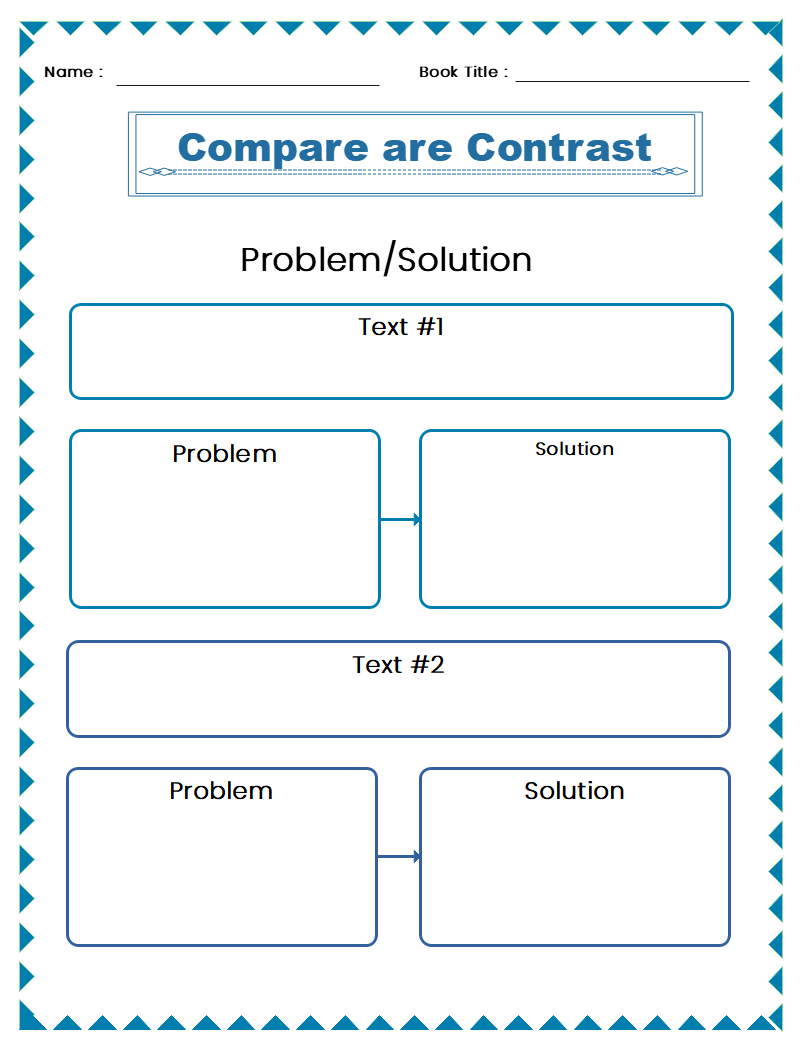Source: EdrawMax Online Edit Now

##### Example 7: Problem and Solution Worksheets

The next example on our list is the Problem and Solution Worksheet. The worksheet is an activity for students and is not exactly a template that can be used freely for any other situation, circumstance, or problem. In this worksheet, there are four sections, each of which contains a certain scenario. In each section, the problem is required and then the solution.

This activity is beneficial for teaching children about problem and solution organizers and the basics of problem-solving. Although the scenario in each section has been explicitly mentioned, it is still required of the students to point out which part is the problem and which part is the solution. This improves their identification skills.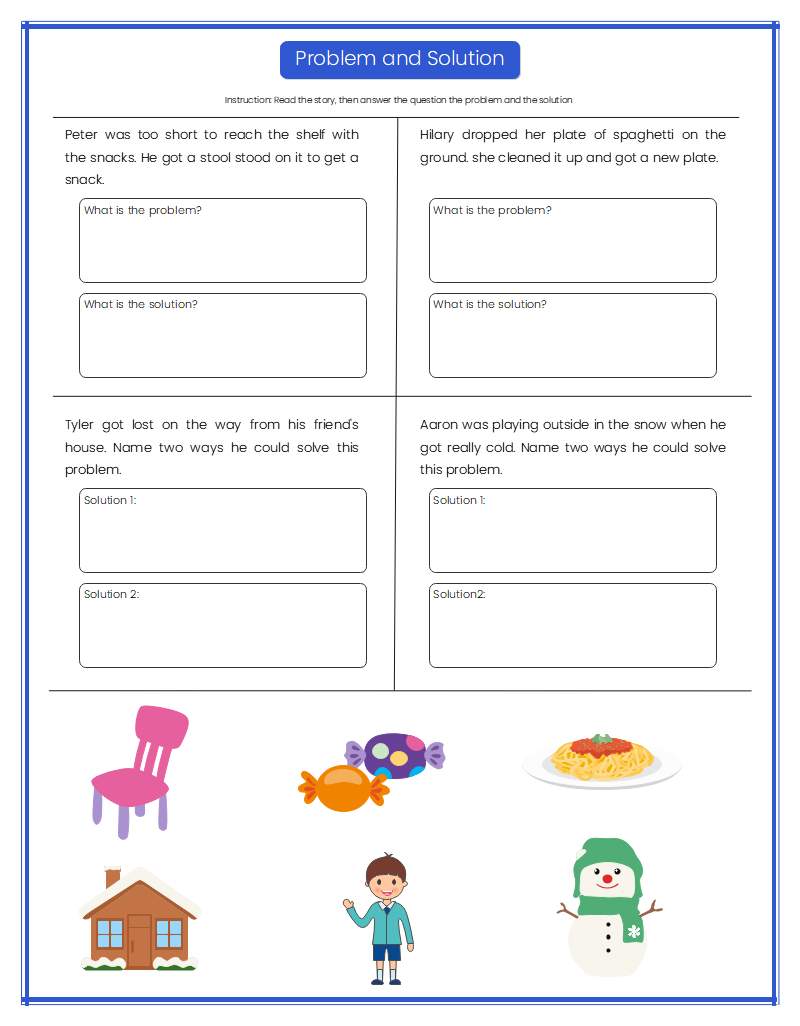Source: EdrawMax Online Edit Now

##### Example 8: Problem and Solution Reading Comprehension Worksheet

This worksheet is also a suitable example to include in this list. The purpose of this worksheet is to tell students to find problems or issues in the story’s character and to list those issues along with their possible solutions.

Albeit with specific instruction, this worksheet also falls in the category of problem and solution graphic organizers. There are three different problems that need to be identified. Three solutions need to be given for each respective problem.

This example has a simple layout unlike some of the more complicated ones we have seen on this list. The worksheet simply requires the students to point out the problem and to suggest a solution. No alternatives are explored, nor are any pros and cons mentioned.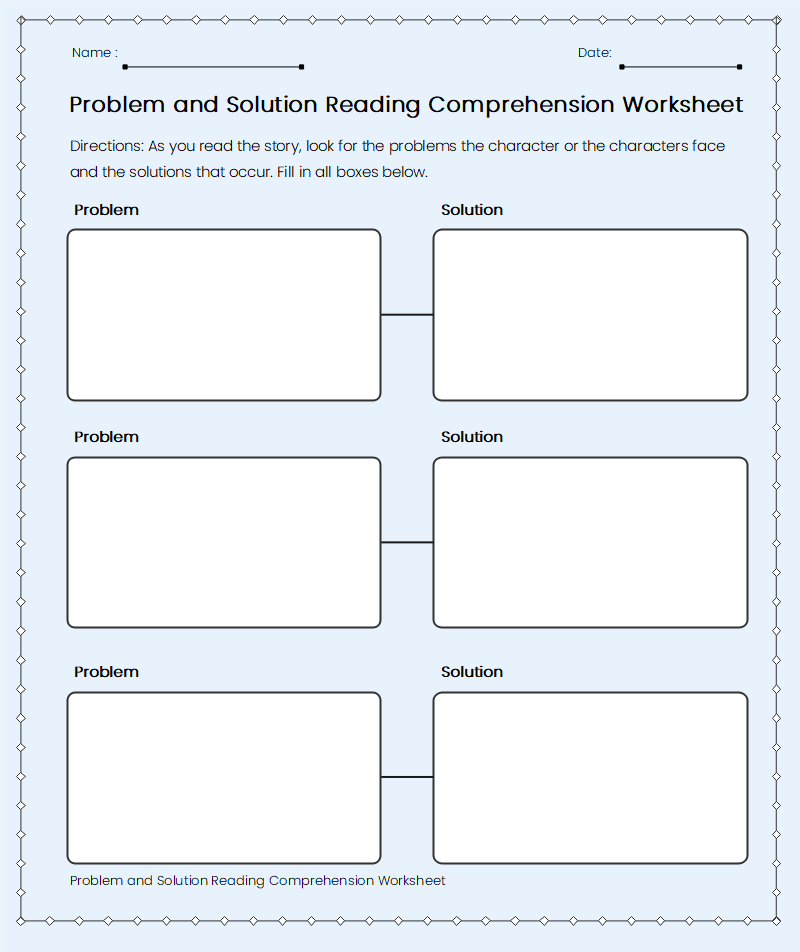Source: EdrawMax Online Edit Now

##### Example 9: Story Elements - Problem and Solution worksheet

This worksheet does not fall in the strict category of graphic organizers. This is a class activity that requires the student to match the problems to suitable solutions. In this example, the problems are given on the left hand and the solutions are given on the right. The solutions and problems are written all jumbled up and are not written adjacent to one another.

This sort of worksheet can be a useful tool in teaching kids the concept behind problem-solving. This example can be a stepping stone using which the students can, later on, realize how they have to present a solution to any problem that they face.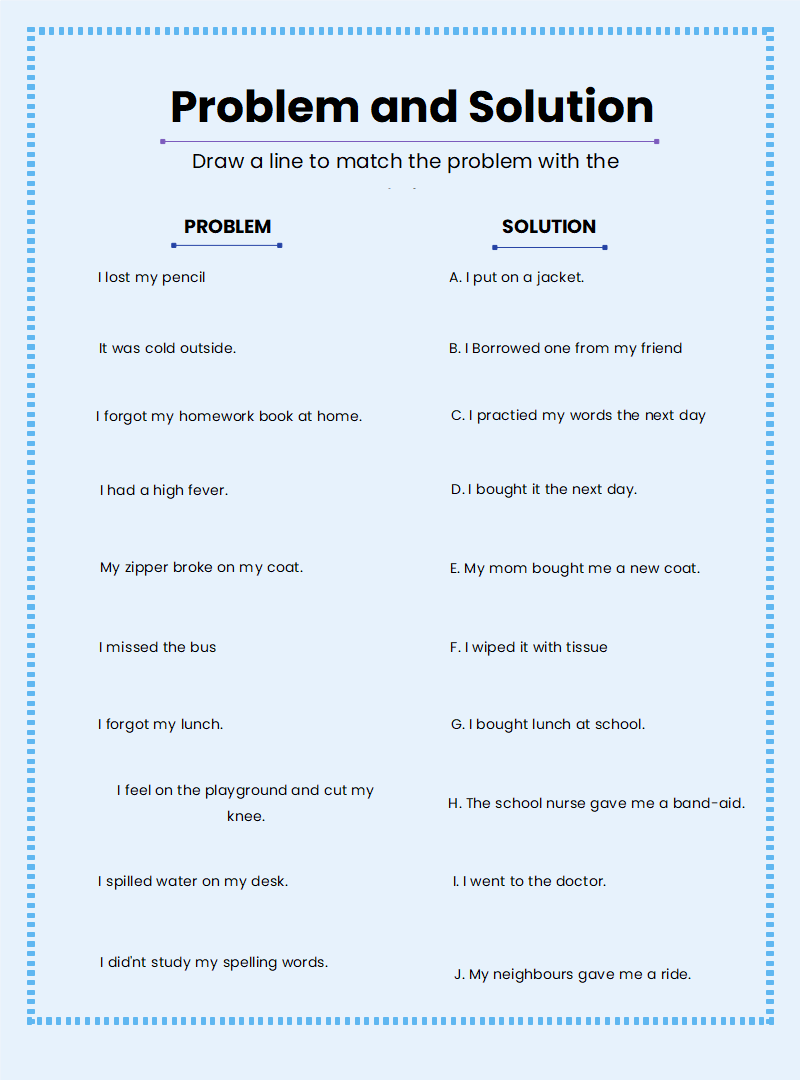Source: EdrawMax Online Edit Now

## 3. Online Problem and Solution Graphic Organizer Maker

Now that we have some idea of what these organizers are, let’s get to the part where we actually make one. If you are looking to make an organizer similar to the examples we saw above, simply head over to EdrawMax Online. At EdrawMax Online, there is a large variety of charts, drawings, and diagrams that you can make. The software is totally online, and it has a user-friendly interface. Shapes can be added from the library by simply dragging them over to the desired spot on the canvas. If you don’t want to make a layout from scratch, you can go to the template gallery and pick a pre-made organizer to edit as your own.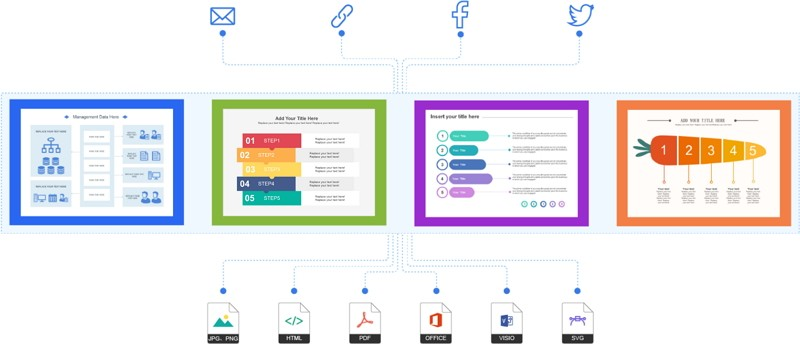Why Choose EdrawMax Online

• Create over 280 diagram types
• Supports team collaboration
• Personal cloud & data protection